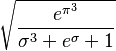# User:Vipul/Sandbox$\sqrt{\frac{e^{\pi^3}}{\sigma^3 + e^\sigma + 1}}$